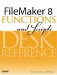# VarianceP()

Category: Aggregate

 Syntax: VarianceP ( field {; field...} )

Parameters:

fieldAny related field, repeating field, or set of non-repeating fields that represent a collection of numbers.

Data type returned: Number

Description:

Returns the variance of a population represented by the non-blank values in the parameter list. Variance of population is a statistical measure of how spread out a set of values is.

The StDevP() of a set of numbers is the square root of the VarianceP() of the set.

Examples:

The variance of a population represented by a set of numbers can be calculated by summing the squares of the distance of each value from the mean, then dividing by n, where n is the number of values.

For instance, given the set of numbers 8, 10, and 12, the mean of the set is 10. The distance of each value from the mean is -2, 0, and 2. The squares of these distances are 4, 0, and 4, and the sum of the squares is 8. The VarianceP is 8 divided by (3), which is 2.67.

``` VarianceP (8; 10; 12)
```

Returns 2.67.

``` VarianceP (7; 11; 15)
```

Returns 10.66.FileMaker 8 Functions and Scripts Desk Reference
ISBN: 0789735113
EAN: 2147483647
Year: 2004
Pages: 352